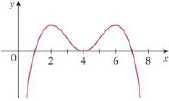Chapter 4.3, Problem 7E### Single Variable Calculus: Early Tr...

8th Edition
James Stewart
ISBN: 9781305270343

#### Solutions

Chapter
Section### Single Variable Calculus: Early Tr...

8th Edition
James Stewart
ISBN: 9781305270343
Textbook Problem

# In each part state the x-coordinates of the inflection points of f. Give reasons for your answers.(a) The curve is the graph of f.(b) The curve is the graph of f′.(c) The curve is the graph of f″.(a)

To determine

To state: The x-coordinates of the inflexion point of f.

Explanation

Given:

The given curve is the graph of f.

Definition used: Inflection point

A point P on a curve under the function f(x) is called an inflection point if f is continuous and curve changes from concave upward to concave downward or from concave downward to concave upward at P.

Reason:

Since the curve is the graph of f and from the given graph, it i

(b)

To determine

To state: The x-coordinates of the inflexion point of f.

(c)

To determine

To state: The x-coordinates of the inflexion point of f.

### Still sussing out bartleby?

Check out a sample textbook solution.

See a sample solution

#### The Solution to Your Study Problems

Bartleby provides explanations to thousands of textbook problems written by our experts, many with advanced degrees!

Get Started

#### Find the median for the following set of scores: 1, 9, 3, 6, 4, 3, 11, 10

Essentials of Statistics for The Behavioral Sciences (MindTap Course List)

#### Evaluate the integrals in Problems 7-36. Check your results by differentiation. 28.

Mathematical Applications for the Management, Life, and Social Sciences

#### Are ABC and DEF congruent? Exercises 7, 8

Elementary Geometry For College Students, 7e

#### Using for |x| < 1,

Study Guide for Stewart's Multivariable Calculus, 8th